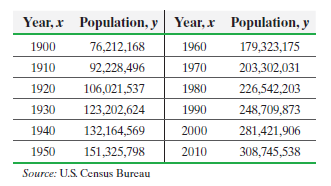×
Get Full Access to Statistics: Informed Decisions Using Data - 5 Edition - Chapter 14.1 - Problem 22
Get Full Access to Statistics: Informed Decisions Using Data - 5 Edition - Chapter 14.1 - Problem 22

×

# ?The U.S. Population The following data represent the population of the United States for the years 1900–2010 An ecologist iISBN: 9780134133539 240

## Solution for problem 22 Chapter 14.1

Statistics: Informed Decisions Using Data | 5th Edition

• Textbook Solutions
• 2901 Step-by-step solutions solved by professors and subject experts
• Get 24/7 help from StudySoup virtual teaching assistantsStatistics: Informed Decisions Using Data | 5th Edition

4 5 1 415 Reviews
14
5
Problem 22

The U.S. Population The following data represent the population of the United States for the years 1900–2010An ecologist is interested in finding an equation that describes the population of the United States over time.

(a) Determine the least-squares regression equation, treating year as the explanatory variable.

(b) A normal probability plot of the residuals indicates that the residuals are approximately normally distributed. Test whether a linear relation exists between year and population.

(c) Draw a scatter diagram, treating year as the explanatory variable.

(d) Plot the residuals against the explanatory variable, year.

(e) Does a linear model seem appropriate based on the scatter diagram and residual plot? (Hint: See Section 4.3.)

(f) What is the moral?

Step-by-Step Solution:

Step 1 of 5) The U.S. Population The following data represent the population of the United States for the years 1900–2010 An ecologist is interested in finding an equation that describes the population of the United States over time. (a) Determine the least-squares regression equation, treating year as the explanatory variable. (b) A normal probability plot of the residuals indicates that the residuals are approximately normally distributed. Test whether a linear relation exists between year and population. (c) Draw a scatter diagram, treating year as the explanatory variable. (d) Plot the residuals against the explanatory variable, year. (e) Does a linear model seem appropriate based on the scatter diagram and residual plot (Hint: See Section 4.3.) (f) What is the moral

Step 2 of 2

## Discover and learn what students are asking

Calculus: Early Transcendental Functions : Evaluating Limits Analytically
?Finding a Limit In Exercises 5-36, find the limit. $$\lim _{x \rightarrow 2} x^{3}$$

Unlock Textbook Solution